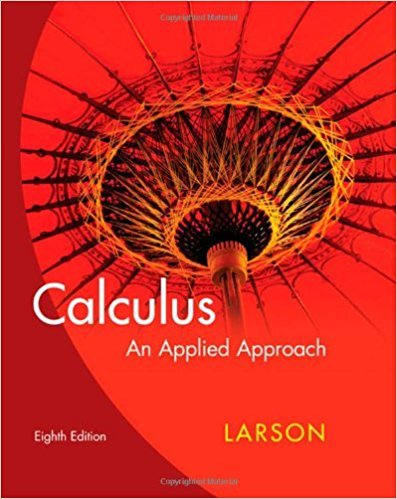×
×

# Solutions for Chapter 2.5: The Chain Rule## Full solutions for Calculus: An Applied Approach | 8th Edition

ISBN: 9780618958252Solutions for Chapter 2.5: The Chain Rule

Solutions for Chapter 2.5
4 5 0 255 Reviews
31
0
##### ISBN: 9780618958252

Since 81 problems in chapter 2.5: The Chain Rule have been answered, more than 21856 students have viewed full step-by-step solutions from this chapter. This expansive textbook survival guide covers the following chapters and their solutions. Chapter 2.5: The Chain Rule includes 81 full step-by-step solutions. This textbook survival guide was created for the textbook: Calculus: An Applied Approach , edition: 8. Calculus: An Applied Approach was written by and is associated to the ISBN: 9780618958252.

Key Calculus Terms and definitions covered in this textbook
• Angle of depression

The acute angle formed by the line of sight (downward) and the horizontal

• Arc length formula

The length of an arc in a circle of radius r intercepted by a central angle of u radians is s = r u.

• Basic logistic function

The function ƒ(x) = 1 / 1 + e-x

• Combinatorics

A branch of mathematics related to determining the number of elements of a set or the number of ways objects can be arranged or combined

• Fibonacci numbers

The terms of the Fibonacci sequence.

• Geometric sequence

A sequence {an}in which an = an-1.r for every positive integer n ? 2. The nonzero number r is called the common ratio.

• Independent variable

Variable representing the domain value of a function (usually x).

• Linear inequality in x

An inequality that can be written in the form ax + b < 0 ,ax + b … 0 , ax + b > 0, or ax + b Ú 0, where a and b are real numbers and a Z 0

• Linear programming problem

A method of solving certain problems involving maximizing or minimizing a function of two variables (called an objective function) subject to restrictions (called constraints)

• Linear regression line

The line for which the sum of the squares of the residuals is the smallest possible

• Nappe

See Right circular cone.

• Order of magnitude (of n)

log n.

• Ordered pair

A pair of real numbers (x, y), p. 12.

• Parameter

See Parametric equations.

• Projection of u onto v

The vector projv u = au # vƒvƒb2v

The graph in three dimensions of a seconddegree equation in three variables.

• Quotient rule of logarithms

logb a R S b = logb R - logb S, R > 0, S > 0

• Real number line

A horizontal line that represents the set of real numbers.

• Recursively defined sequence

A sequence defined by giving the first term (or the first few terms) along with a procedure for finding the subsequent terms.

• Triangular number

A number that is a sum of the arithmetic series 1 + 2 + 3 + ... + n for some natural number n.

×